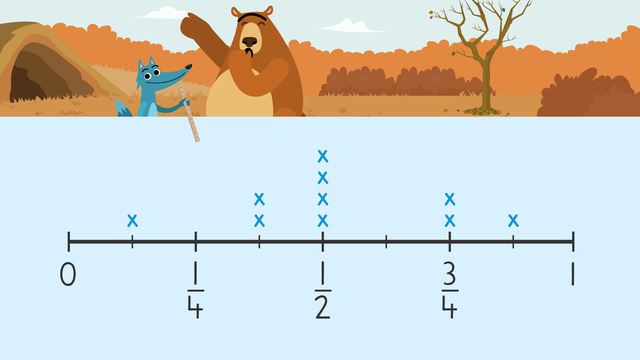# Fractions on Line PlotsRate this video

Ø 5.0 / 2 ratings

The authorTeam Digital
Fractions on Line Plots
CCSS.MATH.CONTENT.4.MD.B.4

## Information about the videoFractions on Line Plots

### Contents

#### What are Line Plots with Fractions?

Line plots with fractions are a type of graph that shows data on a fractional number line. There are x’s created above the line to indicate the frequency of each unit of data.

#### In this Video

Nari is trying to prove to Gus that there will be plenty of snow this upcoming winter. He has recorded the days that have had snow accumulation and put the data in a line plot. We will use the line plots with fraction 4th grade to answer questions about the information. Will Gus believe it snowed?

#### Example of 4th Grade Line Plots with Fractions

Using Line Plots we can answer questions about the data.#### Follow Up Activities for Creating Line Plots with Fractions

There will be additional activities with line plots with fractions worksheet and exercises. These line plots with fractions 4th-grade worksheets will provide students with continued practice using line plots to record data and answer questions about the fractional data.